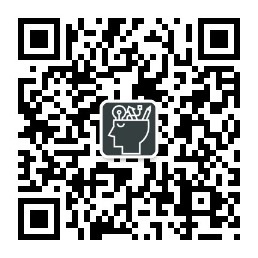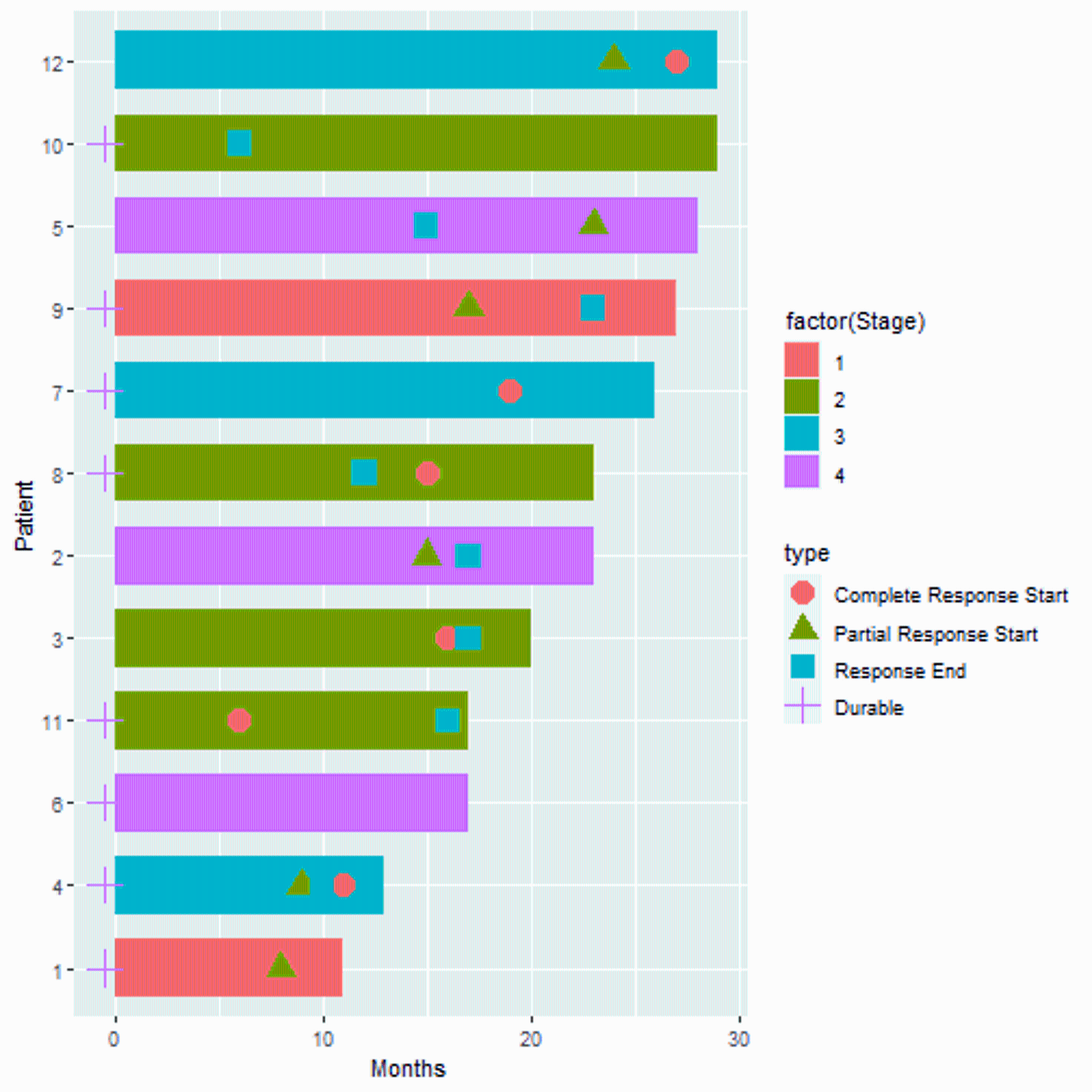V1

2022/09/15阅读：212主题：自定义主题1

# R语言可视化肿瘤领域的泳道图

• 完全缓解（CR, complete response）：所有靶病灶消失，无新病灶出现，且肿瘤标志物正常，至少维持4周。
• 部分缓解（PR, partial response）：靶病灶最大径之和减少≥30%，至少维持4周。
• 疾病进展（PD, progressive disease）：靶病灶最大径之和至少增加≥20%，或出现新病灶。
• 疾病稳定（SD, stable disease）：靶病灶最大径之和缩小未达PR，或增大未达PD。

``suppressMessages(library(tidyverse))set.seed(200)df <- data.frame(Patient = 1:12,                  Months = sample(6:30, 12, replace=TRUE),                 Stage = sample(1:4, 12, replace=TRUE),                 Continued = sample(0:1, 12, replace=TRUE))df <- df |>   mutate(Patient = fct_reorder(factor(Patient), Months)) |> # 画图时保证顺序  group_by(Patient) |>   mutate('Complete Response Start'=sample(c(6:(max(Months)-1),NA), 1,                          prob=c(rep(1, length(6:(max(Months)-1))),5), replace=TRUE),         'Partial Response Start'=sample(c(6:(max(Months)-1),NA), 1,                         prob=c(rep(1, length(6:(max(Months)-1))),5), replace=TRUE),         'Durable'=sample(c(-0.5,NA), 1, replace=TRUE),         'Response End'=sample(c(6:(max(Months)-1),NA), 1,                         prob=c(rep(1, length(6:(max(Months)-1))),5), replace=TRUE)         )# 数据大概这样head(df)## # A tibble: 6 × 8## # Groups:   Patient ##   Patient Months Stage Continued Complete Response Sta…¹ Parti…² Durable Respo…³##   <fct>    <int> <int>     <int>                   <int>   <int>   <dbl>   <int>## 1 1           11     1         1                      10       8    -0.5      NA## 2 2           23     4         1                      NA      15    NA        17## 3 3           20     2         0                      16      NA    NA        17## 4 4           13     3         0                      11       9    -0.5      10## 5 5           28     4         0                      NA      23    NA        15## 6 6           17     4         0                      NA      NA    -0.5      NA## # … with abbreviated variable names ¹​`Complete Response Start`,## #   ²​`Partial Response Start`, ³​`Response End```

``p <- ggplot(df, aes(Months, Patient))+  geom_bar(stat = "identity", aes(fill=factor(Stage)),width = 0.7)p``

xxxxxxxxxx xxxxxxxxxxxx xxxxxxxxxxxxxxx

``df_long <- df |>   pivot_longer(5:8,names_to = "type",values_to = "value") |>   mutate(type=factor(type, # 顺便给type排个序，画图保证顺序                     levels = c("Complete Response Start","Partial Response Start","Response End","Durable")))head(df_long)## # A tibble: 6 × 6## # Groups:   Patient ##   Patient Months Stage Continued type                    value##   <fct>    <int> <int>     <int> <fct>                   <dbl>## 1 1           11     1         1 Complete Response Start  10  ## 2 1           11     1         1 Partial Response Start    8  ## 3 1           11     1         1 Durable                  -0.5## 4 1           11     1         1 Response End             NA  ## 5 2           23     4         1 Complete Response Start  NA  ## 6 2           23     4         1 Partial Response Start   15``

``p1 <- p +   geom_point(data = df_long, aes(value, Patient, shape=type, color=type), size=5)p1``这是这个图已经具有雏形了，但是还少一个箭头，由于R默认的形状（pch）中并没有箭头，所以一开始并没有把箭头表示的信息也加入到`type`这一列中。。

``p2 <- p1 +   geom_segment(data = df |> dplyr::filter(Continued == 1),                         aes(x=Months+0.1, xend=Months+1.5, y=Patient, yend=Patient),                        size = 2,                         arrow = arrow(length = unit(0.3,"cm"))                        )p2``

xxxxxxxxxxxxxxxx坐标轴 xxxxxxxxxxxxxxxxx图例

• 调整大小
• 修改图例形状
• 修改图例标题
• 修改横纵坐标
• 修改整体背景
• ......
``p3 <- p2 +   scale_shape_manual(values = c(16:18,15)) +  scale_color_manual(values = c("red","blue","grey","black"))+  scale_x_continuous(limits = c(-1,32), breaks = -1:30,expand = c(0,0.6))+  labs(fill = "Disease Stage", shape = NULL, color = NULL)+  theme_bw()+  theme(panel.grid.minor=element_blank(),        panel.grid.major=element_blank(),        axis.text.y=element_blank(),        axis.ticks.y=element_blank(),        legend.position = c(0.9,0.3)        )p3``V1